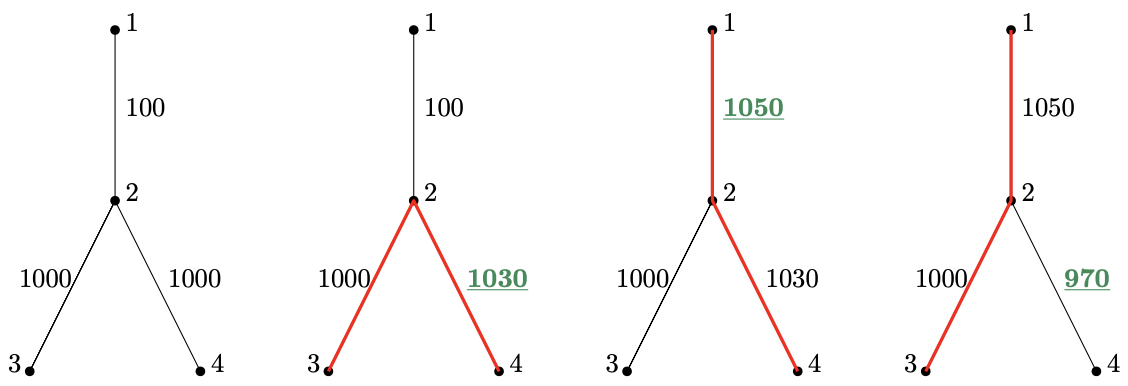시간 제한메모리 제한제출정답맞힌 사람정답 비율
5 초 512 MB205975236.111%

## 문제

You are given a weighted undirected tree on n vertices and a list of q updates. Each update changes the weight of one edge. The task is to output the diameter of the tree after each update.

(The distance between two vertices is the sum of the weights on the unique simple path that connects them. The diameter is the largest of all those distances.)

## 입력

The first line contains three space-separated integers n, q and w (2 ≤ n ≤ 100, 000, 1 ≤ q ≤ 100, 000, 1 ≤ w ≤ 20, 000, 000, 000, 000) – the number of vertices in the tree, the number of updates and the limit on the weights of edges. The vertices are numbered 1 through n.

Next, n − 1 lines describing the initial tree follow. The i-th of these lines contains three space-separated integers ai, bi, ci (1 ≤ ai, bi ≤ n, 0 ≤ ci < w) meaning that initially, there is an edge between vertices ai and bi with weight ci. It is guaranteed that these n − 1 lines describe a tree.

Finally, q lines describing queries follow. The j-th of these lines contains two space-separated integers dj, ej (0 ≤ dj < n − 1, 0 ≤ ej < w). These two integers are then transformed according to the following scheme:

• d'j = (dj + last) mod (n − 1)
• e'j = (ej + last) mod w

where last is the result of the last query (initially last = 0). Tuple (d'j , e'j) represents a query which takes the d'j + 1-th edge from the input and sets its weight to e'j.

## 출력

Output q lines. For each i, line i should contain the diameter of the tree after the i-th update.

## 서브태스크

번호배점제한
111

n, q ≤ 100 and w ≤ 10, 000

213

n, q ≤ 5, 000 and w ≤ 10, 000

37

w ≤ 10, 000 and the edges of the tree are exactly all valid edges of the form {1, i} (Hence, the tree is a star centered at vertex 1.)

418

w ≤ 10, 000, and the edges of the tree are exactly all valid edges of the forms {i, 2i} and {i, 2i + 1} (Hence, if we were to root the tree at vertex 1, it would be a balanced binary tree.)

524

It is guaranteed that after each update a longest simple path goes through vertex 1

627

## 예제 입력 1

4 3 2000
1 2 100
2 3 1000
2 4 1000
2 1030
1 1020
1 890


## 예제 출력 1

2030
2080
2050


## 예제 입력 2

10 10 10000
1 9 1241
5 6 1630
10 5 1630
2 6 853
10 1 511
5 3 760
8 3 1076
4 10 1483
7 10 40
8 2051
5 6294
5 4168
7 1861
0 5244
6 5156
3 3001
8 5267
5 3102
8 3623


## 예제 출력 2

6164
7812
8385
6737
6738
7205
6641
7062
6581
5155


## 힌트

The first sample is depicted in the figure below. The left-most picture shows the initial state of the graph. Each following picture depicts the situation after an update. The weight of the updated edge is painted green, and the diameter is red.The first query changes the weight of the 3rd edge, i.e. {2, 4}, to 1030. The largest distance between any pair of vertices is 2030 – the distance between 3 and 4.

As the answer is 2030, the second query is

d'2 = (1 + 2030) mod 3 = 0

e'2 = (1020 + 2030) mod 2000 = 1050

Hence the weight of the edge {1, 2} is changed to 1050. This causes the pair {1, 4} to be the pair with the greatest distance, namely 2080.

The third query is decoded as

d'3 = (1 + 2080) mod 3 = 2

e'3 = (890 + 2080) mod 2000 = 970

As the weight of the edge {2, 4} decreases to 970, the most distant pair is suddenly {1, 3} with 2050.

## 채점 및 기타 정보

• 예제는 채점하지 않는다.